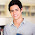Calculating marginal and total opportunity costs. - FreeEconHelp.com, Learning Economics... Solved!

## 9/23/11

This post goes over a question recently asked about calculating opportunity costs given information similar to what is available in a PPF.

 Combination Pizza Computer A 0 7 B 7 6 C 13 5 D 18 4 E 22 3 F 25 2 G 27 1 H 28 0

My recommendation for solving these types of problems is to create another table with 4 more columns.  In these columns, you will calculate the difference in amount of pizza, and the difference in the amount of computers.  For example, moving from point A to point B, we see pizza go from 0 units, to 7 units.
So the difference will be 7 (7-0).  For computers we move from 7 units to 6 units, so the difference is 1 unit (7-6).  Fill up these columns with the relevant information from combinations A to H.

Now create two more columns at the end, that calculate the MC (marginal cost/opportunity cost) of Pizza and computers.  You calculate this by dividing the differences in the two columns.  For example, if you want to find the opportunity cost of pizza, moving from point A to point B, then you have to divide the difference in computers (1) by the difference in pizza (7), which gives tells you that the opportunity cost of 1 pizza is 1/7 computers between these combinations.

You can repeat this procedure all the way down the two columns by dividing the differences and then you will have the opportunity cost of both goods between every combination.
 Combination Pizza P. Diff Computer C. Diff MC Pizza MC Comp A 0 7 B 7 7 6 1 1/7 7 C 13 6 5 1 1/6 6 D 18 5 4 1 1/5 5 E 22 4 3 1 ¼ 4 F 25 3 2 1 1/3 3 G 27 2 1 1 ½ 2 H 28 1 0 1 1 1

So the opportunity cost of the 4th computer is going to use the calculation going from point E to D (or information from the E row).  Since the 4th computer required us to give up 4 pizza, we divide 4 (pizza lost) by 1 (computers gained) to get an opportunity cost of 4.  Which is also shown in the E row, under marginal cost of computers.

Also, the total opportunity cost of producing 5 computers, is equal to the individual opportunity cost (or marginal costs) added up.  So in this example we are moving from combination H to combination C (but the way the table is created we stop at D).  So we can add up the individual MC comps for each of these rows and we get 15 (1+2+3+4+5).  Another way to do this is to see that 13 pizza are being produced at point C, while at point H 28 pizza were being produced.  28-13 gives us 15, so 15 pizza are the opportunity cost of producing 5 computers.

1.Garland L. Harris11 November

thanku

2.There is tons of interesting info at this blog. Be sure to check it out

3.Sourcing locally grown vegetables are key to keeping Sweetgreen’s supply chain stocked with the grademiners freshest organic produce. Instead of requesting a certain crop, Ru also prefers to ask what the local farmers are growing instead. This helps eliminate food waste and gives the restaurant’s patron’s new veggies to try.

4.5.